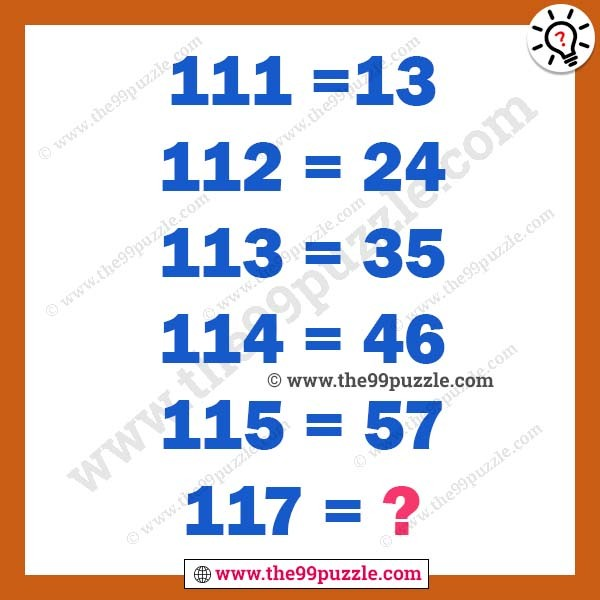# Math logic puzzle for high school students – Puzz222

Math logic puzzle for high school students. Mathematical reasoning puzzles are asked in many exams. These types of puzzles are fun and challenging. How good are you in math? Test your math skills to solve this puzzle. You learn maths shortcut tricks and math logic from it. Sometimes this math puzzle gets very hard but when you crack the math logic this is very easy to solve. In this math equation puzzle, some equations give here. All equations are in the same math logic. You have to crack the logic and solve the last equation.

111=13

112=24

113=35

114=46

115=57

117=?###### Explanation:

111 = 1 [1+1+1] = 13

112= 2 [1+1+2] = 24

113= 3 [1+1+3] = 35

114=4 [1+1+4] = 46

115=5 [1+1+5] = 57

117=7 [1+1+7] = 79